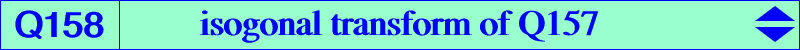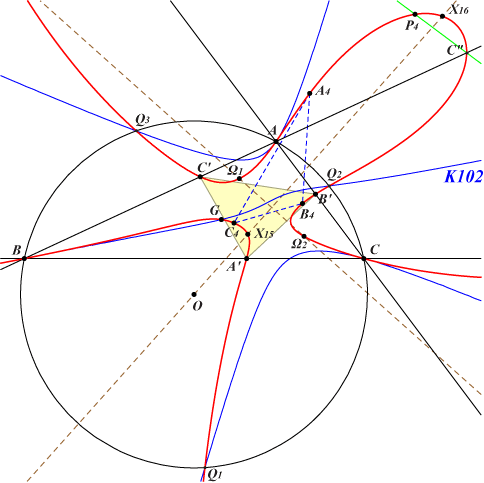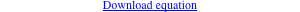∑ x (y - z) [a^4 y z + b^2 c^2 (x^2 + y z)] = 0X(2), X(15), X(16), X(3413), X(3414), X(10221), X(39641), X(39642) Ω1, Ω2 : Brocard points A4, B4, C4 : vertices of the fourth Brocard triangle A', B', C' : vertices of the incentral triangle = cevian triangle of X(1) A", B", C" : traces of the anti-orthic axis on the sidelines of ABC Q1, Q2, Q3 : vertices of the Grebe triangle, see K102 other points belowQ158 is the isogonal transform of Q157. It is a circular quartic with two real asymptotes parallel at X(325) to those of the Kiepert hyperbola and two imaginary asymptotes passing through X(2080). Q158 passes through A, B, C where the tangents are the medians of ABC. These medians meet Q158 again at X(2) and the vertices of the fourth Brocard triangle. Q158 meets the A-bisectors of ABC again at A', A" and two pairs of points , namely : {– a^2 + b^2 + bc : bc : c^2 , – a^2 + c^2 + bc : b^2 : bc} on the internal bisector, {– a^2 + b^2 – bc : – bc : c^2 , – a^2 + c^2 – bc : b^2 : – bc} on the external bisector. These 12 points on the 6 bisectors form 3 groups of 4 points on the perpendicular bisectors of ABC. Q158 meets the anti-orthic axis at A", B", C" (on the sidelines of ABC) and a fourth point P4 on the lines {X2,X36},{X42,X517},{X43,X484},{X44,X513},{X100,X752}, etc, SEARCH = -4.82785658725287. Q158 also contains the three extraversions of P4, on the sidelines of thee incentral triangle. P4 is now X(40109) in ETC. Q158 meets the McCay cubic K003 at A, B, C, X(39641), X(39642) and seven remaining points which are the isogonal conjugates of the Rescassol-Skoubidou points mentioned in page K003. Q158 meets the Euler line at X(2) and three other points also on K019, K511, Q002, Q083, one of them is always real, namely X(10221). Q158 meets the orthocentroidal circle at G, A4, B4, C4, the circular points at infinity, the bicentric pair PU(5). These two latter points lie on the perpendicular bisector (L) of OH. (L) meets Q158 again at two other points on the circum-conic passing through X(2), X(15), X(16) which is the isogonal transform of the Fermat axis.